## Far Off Topic: A Simple Geometry Problem That Had Me "Going in Circles"Started by 5 years ago12 replieslatest reply 5 years ago409 views

At a garage sale recently I bought the book "More Marilyn, Some Like It Bright!" for one U.S. dollar. In that book the following simple geometry problem was posed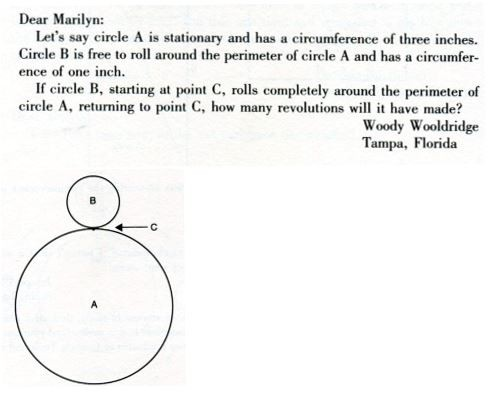The book's author, Marilyn Vos Savant, answered the question. But her answer surprised me so much that I ended up cutting out, and rotating, cardboard circles to prove to myself what was the question's correct answer. Do you know the answer to this simple geometry problem?

[ - ]The obvious answer is 3, but I think you have to add one more because the track on which the circle is moving is itself doing a rotation.

So 4 should be the right answer.

3 would be the answer on a straight track.

[ - ]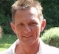That's what I figured out, too.

[ - ]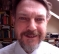To work out the distance traveled you need to consider the motion of the centre of the circle B around A - this gives the travel length as 4 units.

[ - ]what if B  is same size as A? would the answer be 1+1 = 2 ?

[ - ]Yes

[ - ]I am a bit worried now

[ - ][ - ]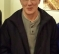Friedman-

That made me laugh.  I don't recall being able to do write-in answers for the SAT, but that sure was one case where it was needed.

-Jeff

[ - ]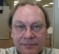I like it. I don't recall ever running across that anywhere until now.

Maybe another way to "see" this is imaging doing what the Egyptians did when using a measuring wheel to build the pyramids, but instead of a perfectly flat surface (ignoring the earth's curvature), if there were intervening bumps along the path, then traversing a bump would have the effect of foreshortening the underlying lineal length.

Another question to ask: is the circle on circle example the worst case for this (i.e. leads to the longest distance)? I'm not into topology, but there may be some equivalence for types of surfaces that do not "fold back" onto themselves. This is easy to see if you imagine rolling over a cube along the path, as the traverse up & down the vertical sides adds nothing to the lineal distance marked.

[ - ]Clearly it is no more than rubbish distortion of the meaning of revolution of circle A.

Rev of 1 of circle A is relative to starting point on surface B.

What is presented here in videos is redefinition of rev 1 as that relative to the observant eye on circle B.

[ - ]